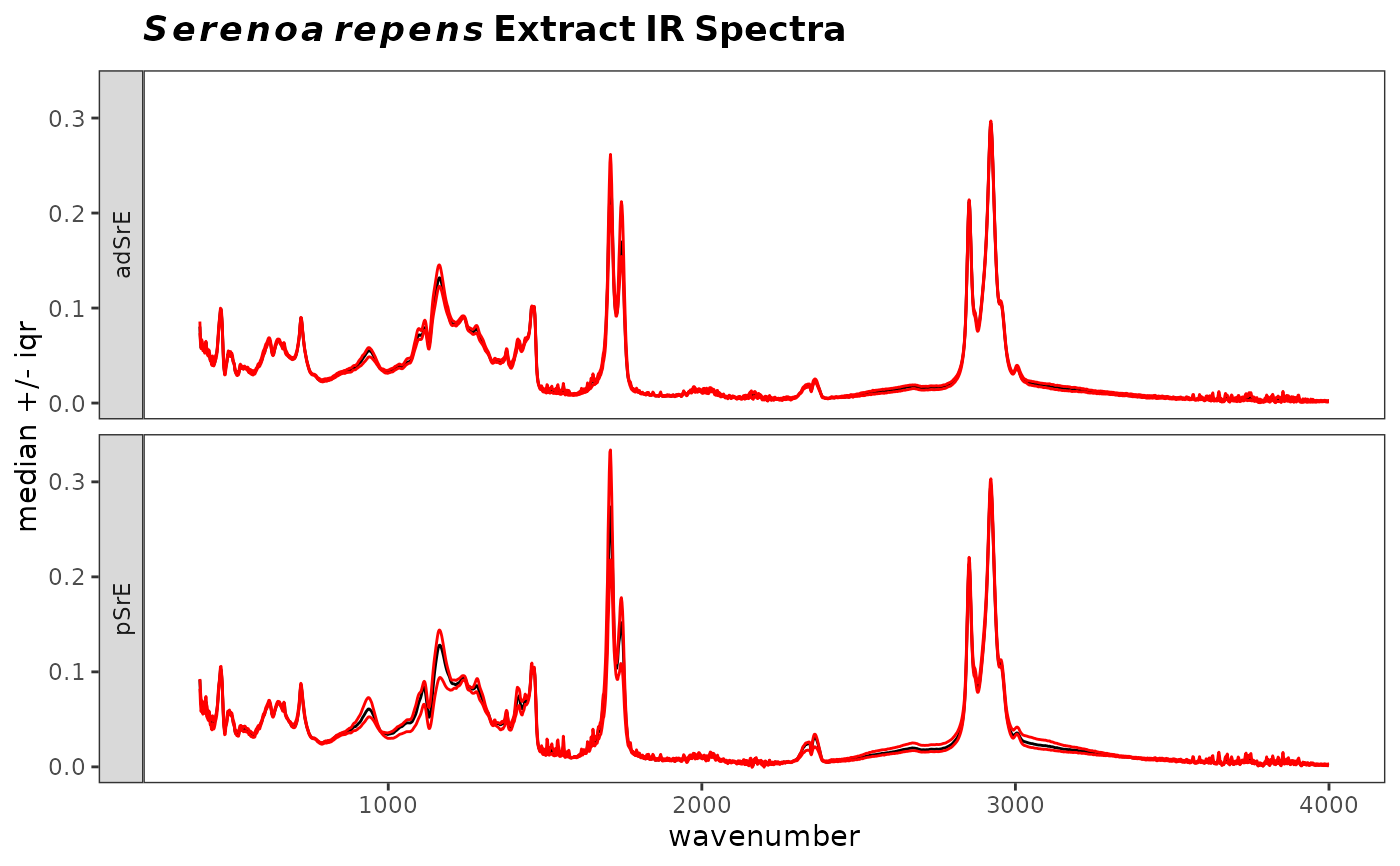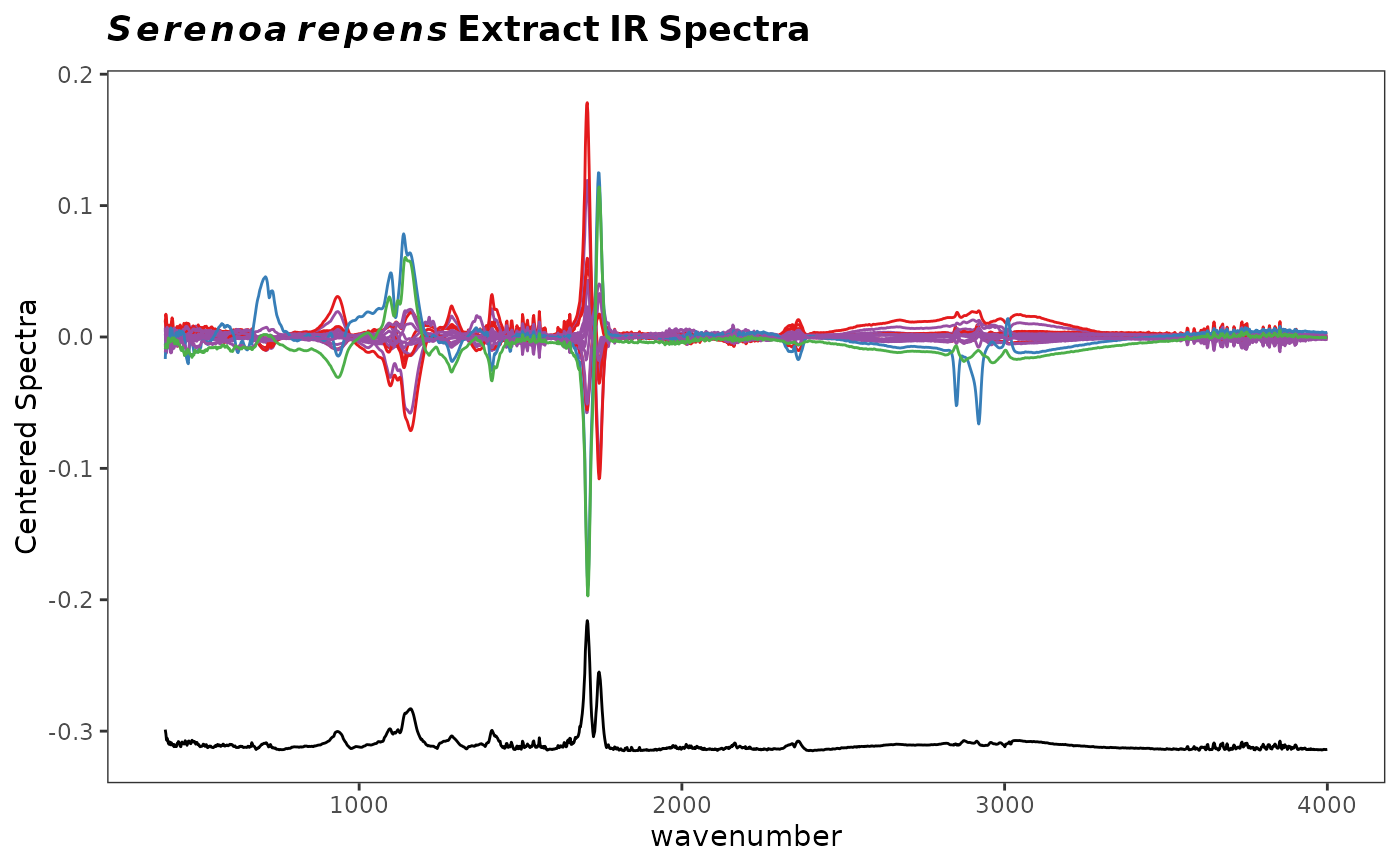Compute and plot various measures of central tendency and spread for a Spectra object. Several different measures/spreads are available. These are useful as an overview of where a data set varies the most.

surveySpectra(
spectra,
method = c("sd", "sem", "sem95", "mad", "iqr"),
by.gr = TRUE,
...
)

surveySpectra2(
spectra,
method = c("sd", "sem", "sem95", "mad", "iqr"),
lab.pos = 0.9 * max(spectra\$freq),
...
)

## Arguments

spectra

An object of S3 class Spectra to be analyzed.

method

Character. One of c("sd", "sem", "sem95", "mad", "iqr").

by.gr

Logical, indicating if the analysis is to be done by group or not. Applies to surveySpectra only.

...

Parameters to be passed to the plotting routines. Applies to base graphics only.

lab.pos

Numeric, giving the frequency where the label should be drawn. Applies to surveySpectra2 only.

## Value

The returned value depends on the graphics option selected (see GraphicsOptions).

base:

None. Side effect is a plot.

ggplot2:

The plot is displayed, and a ggplot2 plot object is returned if the value is assigned. The plot can be modified in the usual ggplot2 manner.

## Details

For surveySpectra the method choice works as follows: sd plots the mean spectrum +/- the standard deviation, sem plots the mean spectrum +/- the standard error of the mean, sem95 plots the mean spectrum +/- the standard error at the 95 percent confidence interval, mad plots the median spectrum +/- the median absolute deviation, and finally, iqr plots the median spectrum + the upper hinge and - the lower hinge.

For surveySpectra2, the spectra are mean centered and plotted. Below that, the relative summary statistic is plotted, offset, but on the same scale.

## Functions

• surveySpectra(): Spectral survey emphasizing mean or median spectrum, optionally by group.

• surveySpectra2(): Spectral survey emphasizing variation among spectra.

## Author

Bryan A. Hanson (DePauw University), Tejasvi Gupta.

## Examples


# This example assumes the graphics output is set to ggplot2 (see ?GraphicsOptions).
library("ggplot2")
data(SrE.IR)
myt <- expression(bolditalic(Serenoa) ~ bolditalic(repens) ~ bold(Extract ~ IR ~ Spectra))

p1 <- surveySpectra(SrE.IR, method = "iqr")
#>
#> Group EPO has 3 or fewer members
#>  so your stats are not very useful...
#>  This group has been dropped for display purposes!
#>
#> Group OO has 3 or fewer members
#>  so your stats are not very useful...
#>  This group has been dropped for display purposes!
p1 <- p1 + ggtitle(myt)
p1p2 <- surveySpectra2(SrE.IR, method = "iqr")
p2 <- p2 + ggtitle(myt)
p2